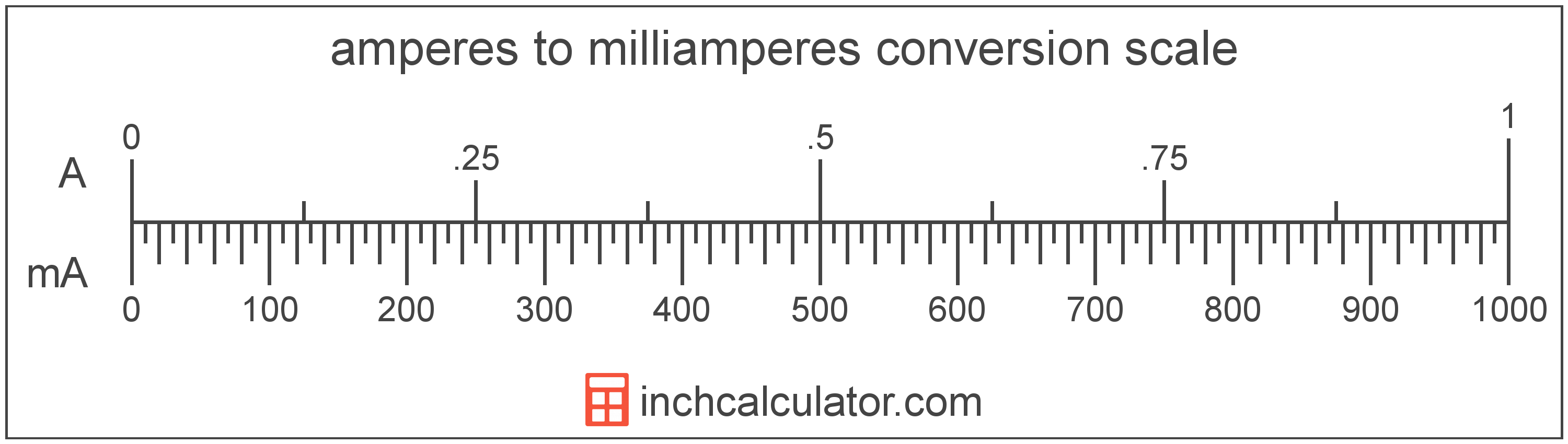# Milliamperes to Amperes Conversion

Enter the electric current in milliamperes below to get the value converted to amperes.

Results in Amperes:1 mA = 0.001 A

## How to Convert Milliamperes to AmperesTo convert a milliampere measurement to an ampere measurement, divide the electric current by the conversion ratio.

Since one ampere is equal to 1,000 milliamperes, you can use this simple formula to convert:

amperes = milliamperes ÷ 1,000

The electric current in amperes is equal to the milliamperes divided by 1,000.

For example, here's how to convert 5,000 milliamperes to amperes using the formula above.
5,000 mA = (5,000 ÷ 1,000) = 5 A

## Milliamperes

One milliampere is equal to 1/1,000 of an ampere, which is the electrical current equal to the flow of one coulomb per second.

The milliampere is a multiple of the ampere, which is the SI base unit for electric current. In the metric system, "milli" is the prefix for 10-3. A milliampere is sometimes also referred to as a milliamp. Milliamperes can be abbreviated as mA; for example, 1 milliampere can be written as 1 mA.

## Amperes

The ampere, commonly referred to as an "amp," is the electrical current constant equal to the flow of one coulomb per second.

The ampere was previously defined as a constant current that when passed through two straight and parallel conductors that are spaced one meter apart, will produce a force equal to 0.0000002 newtons per meter of length.

In 2019, the ampere was redefined as the electric current corresponding to the flow of 1/(1.602 176 634 × 10-19) elementary charges per second.

The ampere is the SI base unit for electric current in the metric system. An ampere is sometimes also referred to as an amp. Amperes can be abbreviated as A; for example, 1 ampere can be written as 1 A.

Ohm's Law states the current between two points on a conductor is proportional to the voltage and inversely proportional to the resistance. Using Ohm's Law, it's possible to express the current in amperes as an expression using resistance and voltage.

IA = VVR

The current in amperes is equal to the potential difference in volts divided by the resistance in ohms.

## Milliampere to Ampere Conversion Table

Milliampere measurements converted to amperes
Milliamperes Amperes
1 mA 0.001 A
2 mA 0.002 A
3 mA 0.003 A
4 mA 0.004 A
5 mA 0.005 A
6 mA 0.006 A
7 mA 0.007 A
8 mA 0.008 A
9 mA 0.009 A
10 mA 0.01 A
20 mA 0.02 A
30 mA 0.03 A
40 mA 0.04 A
50 mA 0.05 A
60 mA 0.06 A
70 mA 0.07 A
80 mA 0.08 A
90 mA 0.09 A
100 mA 0.1 A
200 mA 0.2 A
300 mA 0.3 A
400 mA 0.4 A
500 mA 0.5 A
600 mA 0.6 A
700 mA 0.7 A
800 mA 0.8 A
900 mA 0.9 A
1,000 mA 1 A

## References

1. International Bureau of Weights and Measures, The International System of Units, 9th Edition, 2019, https://www.bipm.org/documents/20126/41483022/SI-Brochure-9-EN.pdf